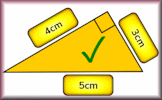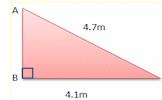# Pythagorean Day

If the square of the day added to the square of the month equals the square of the last two digits of the year then it's a Pythagorean Day.

Example: 24th July 2025 (24² + 7² = 25²)
Also 24/10/2026 and 04/03/2105.## Demonstrating Pythagoras

Short clip from QI: Stephen Fry demonstrates Pythagoras' theorem.

Try It!## Make Them Right

Arrange the measurements next to the sides of the triangles so that each diagram is correct according to Pythagoras' Theorem.

Try It!## Pythagoras

An online exercise to test your understanding of and ability to apply Pythagoras' Theorem.

Try It!## Pythagoras :: All Resources

Have a look at the main Pythagoras Topic page for links to many more activities including Starters, videos and games.

Go!For All: